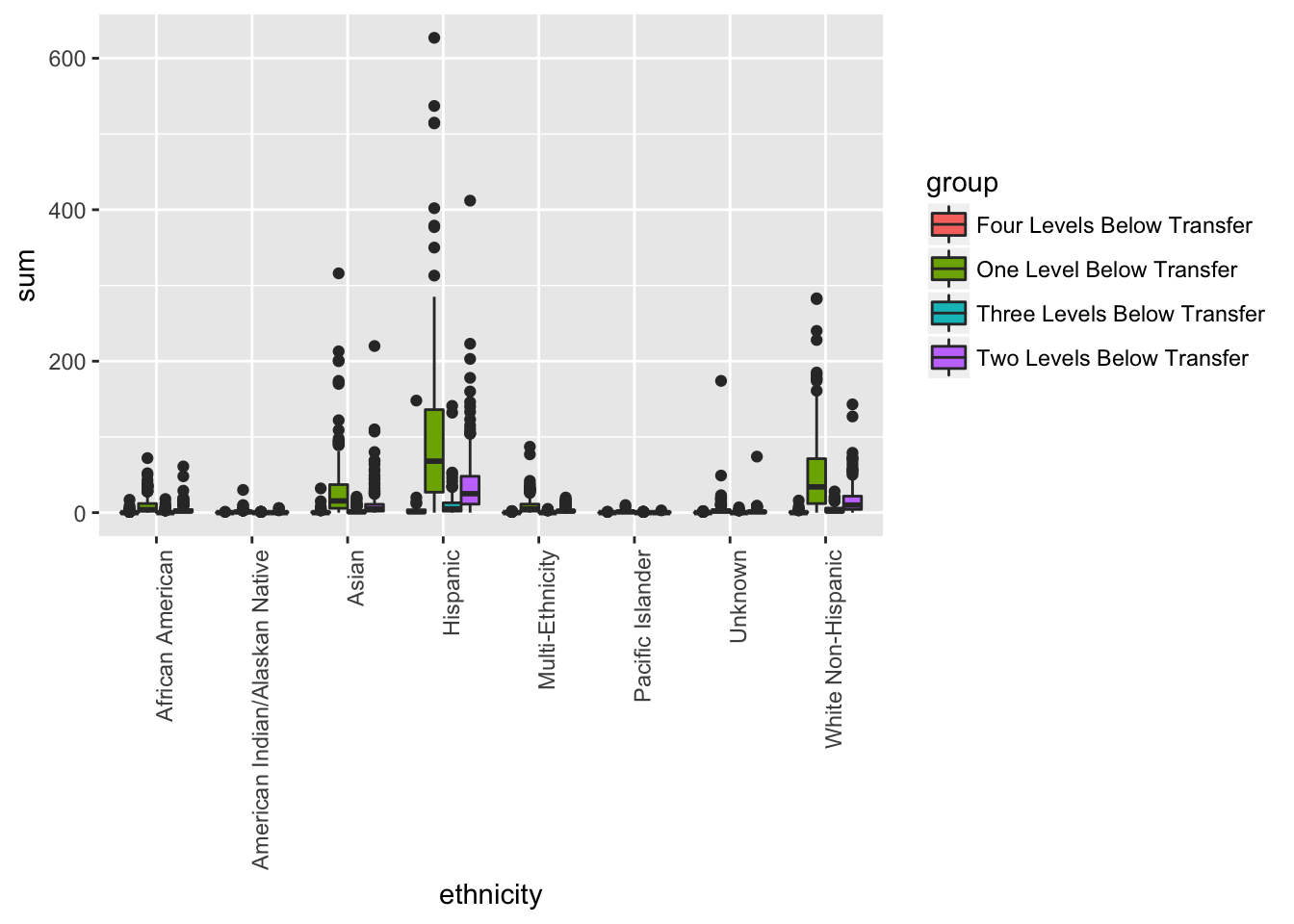# Plotly for College Analysis

Community colleges for many is a stepping stone to a larger dream of four year university. However, many of the risk factors that prevent students from succeeding in school are highly prevelant in the student body of 2-year institutions.

In this post, we explore open college data. This dataset includes the name of the college, the aggregated number of people who passed a particular subject by transfer level and race. The data itself contains information on race, and college name. It also contains information on the pass rate/number of students who passed by subject.

Let’s read our data and take a look:

There is a wonderful package called `DT` that creates interactive plots in HTML. It even comes with a search feature. Check it out.

``````#Load Packages:
require(DT)``````
``## Loading required package: DT``
``require(plotly)``
``## Loading required package: plotly``
``## Loading required package: ggplot2``
``````##
## Attaching package: 'plotly'``````
``````## The following object is masked from 'package:ggplot2':
##
##     last_plot``````
``````## The following object is masked from 'package:stats':
##
##     filter``````
``````## The following object is masked from 'package:graphics':
##
##     layout``````
``school <- read.table(url, header = T)``

## ggplot

The ggplot package is essentially the bread and butter of beautiful and easy to use plots. We will start with `data = school` (duh), and `x = ethnicity, y = sum`. The “sum”" here is just the total number of students who passed given course.

``````g <- ggplot(data = school,
aes(x = ethnicity, y = sum, fill = group)) + geom_boxplot() +
theme(axis.text.x = element_text(angle = 90, hjust = 1)) #Vertical Axis
g``````## Interactivity

In order to make this plot interactive, we have to do something really challenging. Are you ready? Here we go…

Type the following line of code.

``ggplotly(g)``
``````## We recommend that you use the dev version of ggplot2 with `ggplotly()`

That’s it! We can now do all sorts of amazing plots with minimal code! Let’s look as some more stuff.

``````g <- ggplot(data = school,
aes(x = ethnicity, y = success, fill = ethnicity)) +
geom_bar(stat = "identity")  +
theme(axis.text.x = element_text(angle = 90, hjust = 1)) #Vertical Axis
ggplotly(g)``````
``````## We recommend that you use the dev version of ggplot2 with `ggplotly()`

Make sure you clean your data!

``````#Refactor
school\$group <- factor(school\$group,
levels = c("One Level Below Transfer",
"Two Levels Below Transfer",
"Three Levels Below Transfer",
"Four Levels Below Transfer"))

g <- ggplot(data = school,
aes(x = students, y = sum, color = ethnicity)) +
geom_point() + facet_wrap( ~ group, ncol=2)
ggplotly(g)``````
``````## We recommend that you use the dev version of ggplot2 with `ggplotly()`
``````g <- ggplot(data = school,
aes(x = subject, y = sum, color = ethnicity)) +
geom_boxplot() + facet_wrap( ~ ethnicity, ncol=2)
ggplotly(g)``````
``````## We recommend that you use the dev version of ggplot2 with `ggplotly()`
``````g <- ggplot(data = school,
``````## We recommend that you use the dev version of ggplot2 with `ggplotly()`